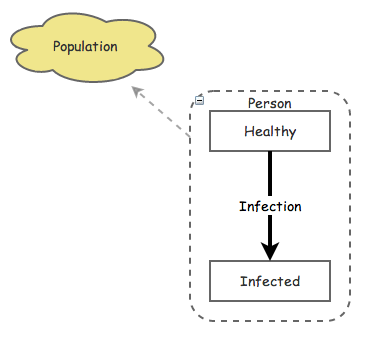# Agent Populations

Agent Populations are a key ingredient in the construction of Agent Based Models. Agent Populations are used to actually store the agents during a simulation. There are two primary factors that control an Agent Population: the size of the population (the number of agents), and the agent base for the population (what type of agent will populate that population).

The population size is the number of agents in the population at the start of the simulation (the size can also be changed dynamically during the simulation by destroying agents or creating new agents).

The agent base defines what types of agents will be contained by the population. Your model can contain one or more definitions of agents (just like it can contain one or more populations of agents), each definition or Agent Base is specified by creating a Folder primitive around the portion of the model that will define that agent. Then the Extension property of the resulting Folder must be set to "Agent". After this is done, the Agent Base property of the Agent Population can be set to the Folder's name.

The following illustration shows what a resulting model might look like after this setup is completed. The Agent Population primitive is Population while the agent base Folder is labeled Person:## Accessing Individual Agents

Insight Maker includes a number of functions to access the individual agents within a population. The simplest of these is the FindAll() function. Given an agent population primitive that we'll call Population, the FindAll function returns a vector containing all the agents within that agent population:

```[Population].FindAll()
```

If your agent population currently contained 100 agents, FindAll would return a vector with 100 elements where the first element referred to the first agent, the second element referred to the second agent, and so on. It is important to note that these elements are agent references, not numbers. So you can use a function like Reverse() on the resulting vector, but you cannot directly use functions like Mean(), as the agent references are not numerical values. We will see how to access the values for agents next.

In addition to the FindAll function, other find functions return a subset of the agents in the model. For instance, the FindState() and FindNotState() functions return, respectively, agents that either have the given state active or not active. For instance, imagine an agent-based disease model where our agents had a state primitive called Infected that represented whether the agent was currently sick. We could get a vector of the agents in our population that were currently sick using the following:

```[Population].FindState([Infected])
```

And we could obtain a vector of the agents that were not currently infected with:

```[Population].FindNotState([Infected])
```

Find functions can also be chained together. For instance, if we added a Male state primitive to our agents to represent whether or not the agent was a man; we could obtain a vector of all currently infected men with something like the following:

```[Population].FindState([Infected]).FindState([Male])
```

Nesting find statements is effectively using Boolean AND logic (like you might use on a search engine: "Infected AND Male"). To perform Boolean OR logic (e.g. "Infected OR Male") and return all the agents that are either infected or a man (or both), you can use the Union function to merge two vectors:

```Union([Population].FindState([Infected]), [Population].FindState([Male]))
```

If you wanted the agents that were either infected or men (but not both simultaneously), you could use:

```Difference([Population].FindState([Infected]), [Population].FindState([Male]))
```

## Agent Values

Once you have a vector of agents, you can extract the values of the specific primitives in those agents using the Value() and SetValue() functions.

The Value function uses two arguments: a vector of agents and the primitive for which you want the value. It returns the value of that primitive in each of the agents. For instance, let's say our agents have a primitive named Height. We could get a vector of the height of all the people in the model like so:

```[Population].FindAll().Value([Height])
```

A vector of heights by itself is generally of not much use. Often we will want to summarize the vector of agents: converting the vector to a single number that represents some property of the population. For instance, we could determine the average height of individuals in the population. The following equation calculates the mean value of agents' height:

```Mean([Population].FindAll().Value([Height]))
```

In addition to determining the value of a primitive in an agent, you can also manually set the agents’ primitive values using the SetValue function. It takes the same arguments as the Value function, in addition to the value to which you want to set primitives. For instance, we could use the following to set the height of all our agents to 2.1:

```[Population].FindAll().SetValue([Height], 2.1)
```

## Creating and Removing Agents

You can programmatically create new agents in an agent population by using the add() function of the agent population. For example, the following will create a new agent that is part of the population My Population:

```[My Population].add()
```

The add() function will return the newly created agent allowing you to configure it. For example:

```newAgent <- [My Population].add()
newAgent.SetLocation({x: 100, y: 50}) # Set the location of the agent
newAgent.SetValue([Age], 72) # Set the agent's [Age] primitive value
```

You can use the remove() function of an agent to remove it from an agent population. This can be thought of as deleting or killing the agent.

## Relationships Between Agents

Each Agent Population contains both a spatial geometry and a network geometry that may be used to relate agents.